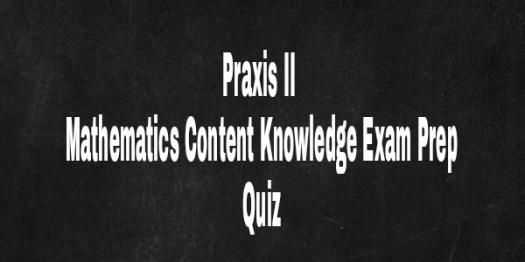# PRAXIS II Mathematics Content Knowledge Exam Prep

10 Questions | Total Attempts: 104SettingsMathematics is all encompassing with various laws and principles to apply. Laws and theorems such as Pythagoras theorem, principles of angle in various positioning, calculus, indices and several others require depth knowledge to accurately apply them in real life. Preparation determines performance, examine your competence by taking this simple quiz.

• 1.
Which of these is alien to trigonometry?
• A.

Law of tangents

• B.

Law of sines

• C.

Law of cosines

• D.

Law of reciprocals

• 2.
What mathematical principle governs sextants?
• A.

Geometry

• B.

Logic

• C.

Statistics

• D.

Trigonometry

• 3.
Which of these is jot a measurement method for angles?
• A.

• B.

Slopians

• C.

Degrees

• D.

• 4.
What is the total angle obtained in a circle?
• A.

157°

• B.

360°

• C.

125°

• D.

145°

• 5.
Which of these is not part of a triangle?
• A.

Congruents

• B.

Opposite

• C.

• D.

Hypotenuse

• 6.
Which of these is not a quadrilateral?
• A.

Rectangle

• B.

Square

• C.

Trapezium

• D.

Triangle

• 7.
Which of these is not a numerical descriptor?
• A.

Median

• B.

Confidence interval

• C.

Standard deviation

• D.

Mean

• 8.
What is the total of internal angles in a quadrilateral?
• A.

180°

• B.

120°

• C.

100°

• D.

90°

• 9.
Which of these is not geometrical element?
• A.

Perimeter

• B.

Length

• C.

Area

• D.

Volume

• 10.
What is classical geometry?
• A.

Differential geometry

• B.

Topology

• C.

Convex geometry

• D.

Euclidean geometry

Related TopicsBack to top